• # include # include using namespace std ; double fun ( double x ) { return pow ( x + 1 , 1.0 ..."第%d次迭代:%.6f\n" , ++ t , r ) ; } return 0 ; }
#include<iostream>
#include<math.h>
using namespace std;

double fun(double x)
{
return pow(x+1,1.0/3);
}

int main()
{
double l = 1,r = 1.5;
int t = 0;
while(fabs(r - l) >= 0.000001)
{
l = r;           //第一次循环中x0
r = fun(r);      //第一次循环中x1
printf("第%d次迭代:%.6f\n",++t,r);
}
return 0;
}




展开全文• 不动点迭代法（Fixed point iteration method） 原理网上已经很多了，这里不再赘述。 需要注意的是不动点迭代法的使用条件。 举个简单的例子，利用不动点法求解方程f(x) = 1 + 0.5*sin(x) − x = 0在（1,2）区间的根...
不动点迭代法（Fixed point iteration method）
原理网上已经很多了，这里不再赘述。
需要注意的是不动点迭代法的使用条件。
举个简单的例子，利用不动点法求解方程f(x) = 1 + 0.5*sin(x) − x = 0在（1,2）区间的根。
令g(x)=1+0.5*sin(x)

g = @(x) fun2(x);
% Initialization
x0 = 0;
tol = 1e-5;
maxIter = 40;

% Test biSection function
[xStar,xRoot] = fixPoint(g,x0,tol,maxIter);
fprintf('The fixed point is: %d\n',xStar);
fprintf('The root of the equation is: %d\n',xRoot);

function [xStar,xRoot] = fixPoint(fun2,x0,tol,maxIter)
% Inputs:
% fun2: a function handle, standing for the function written above
% x0: the initial guess of the fixed point
% tol: the tolerance within which the program can stop
% maxIter: the maximum number of iterations the program is allowed to run
% Outputs:
% xStar: the numerical value of the fixed point
% xRoot: the numerical value of the root

x = zeros(maxIter,1);
x(1) = fun2(x0);
i = 1;

while abs(fun2(x(i))-x(i))>tol && i<maxIter
x(i+1) = fun2(x(i));
xStar = x(i);
i = i+1;
end
xRoot = x(i);

end

function gx = fun2(x)
gx = 1+0.5*sin(x);
end




展开全文matlab 算法
• 【问题描述】在[a,b]区间内寻找方程x**5-2*x-1=0的根的初始近似值位置，确定不动点迭代的初始点（可能有多个），然后使用不动点迭代法求方程的根（可能有多个根）。前后两次迭代的差的绝对值小于delta后停止迭代。 ...计算方法
• Python实现二分法、不动点迭代法、牛顿法 数值分析：Python实现二分法、不动点迭代法、牛顿法 一、问题定义 二、相关概念 三、方程求根的几种常用方法 1、二分法 2、不动点迭代法 3、牛顿法 题目：编写实现...
Python实现二分法、不动点迭代法、牛顿法
数值分析：Python实现二分法、不动点迭代法、牛顿法
一、问题定义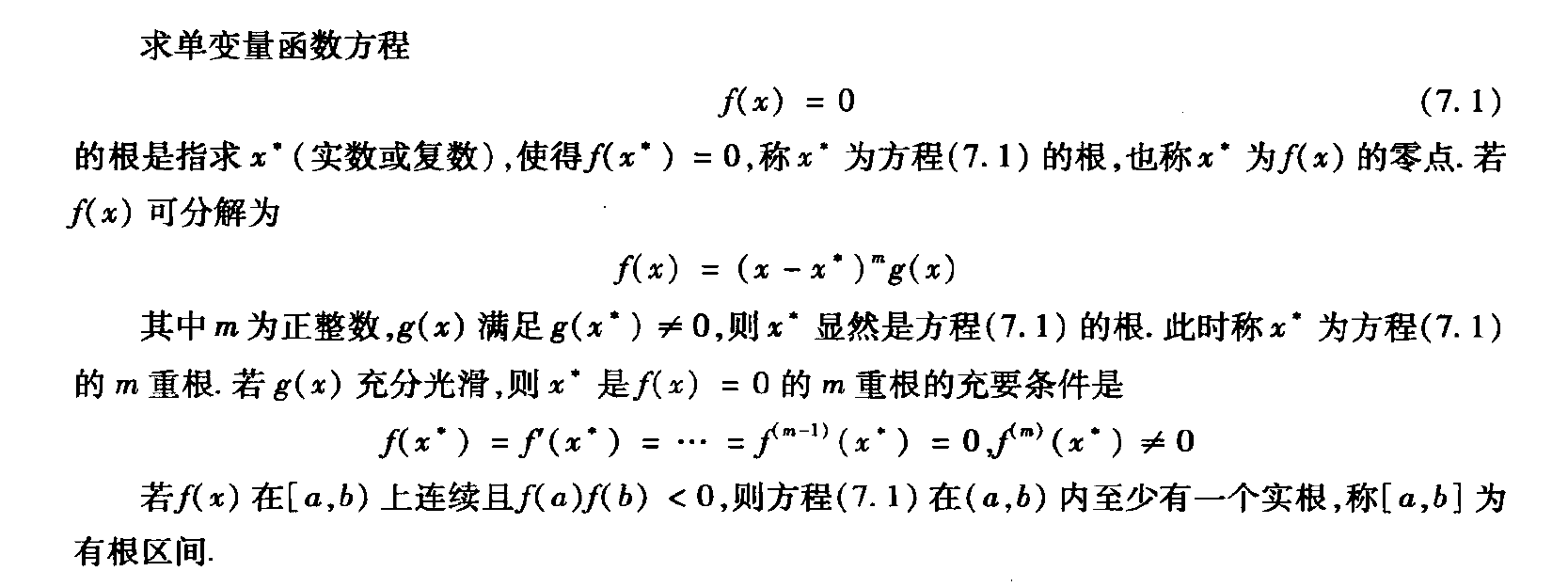二、相关概念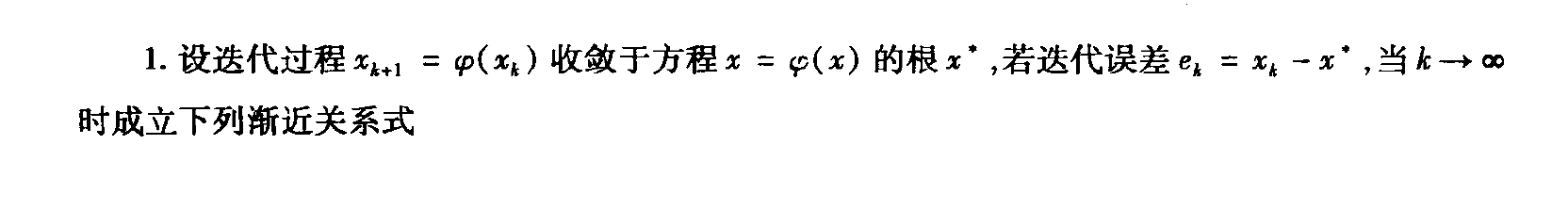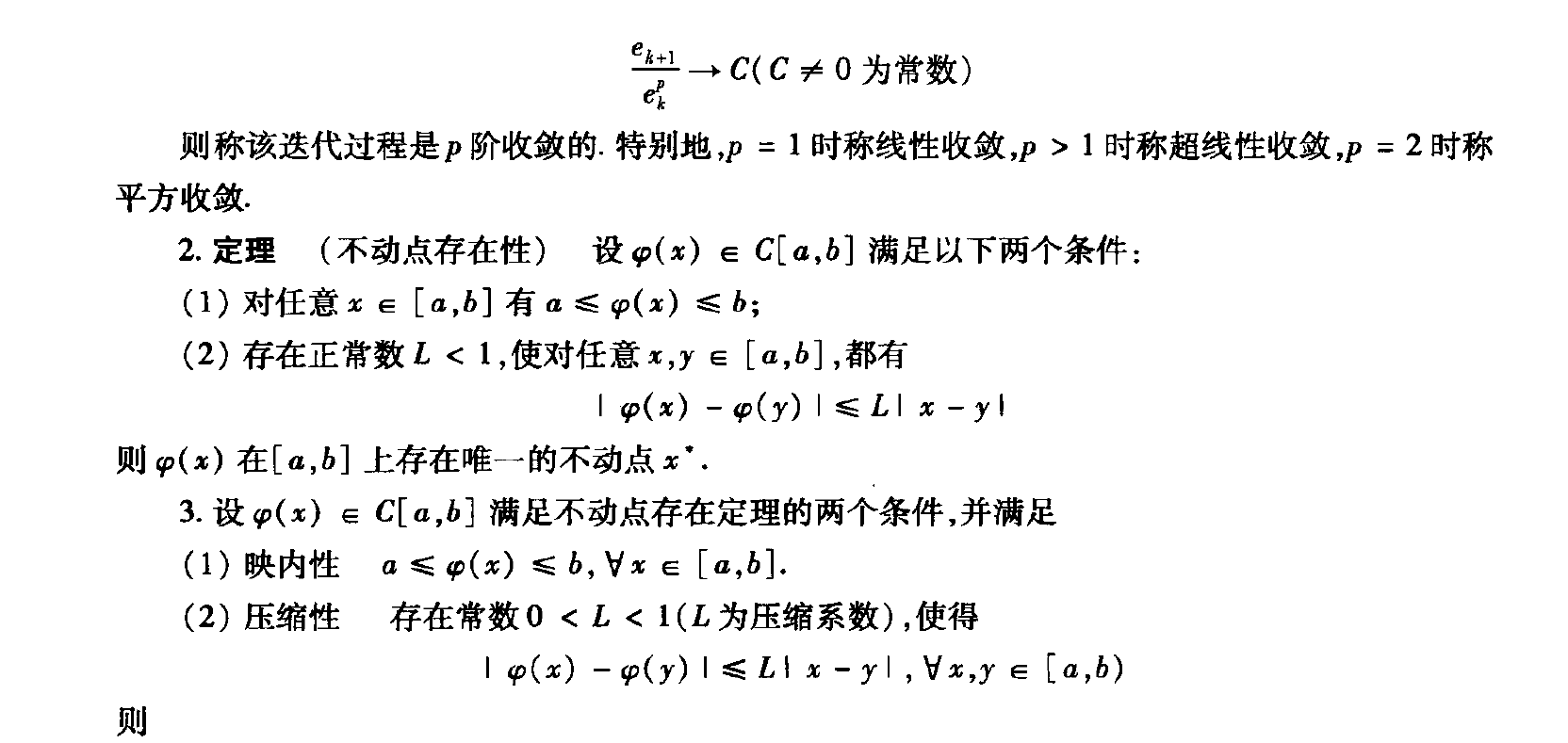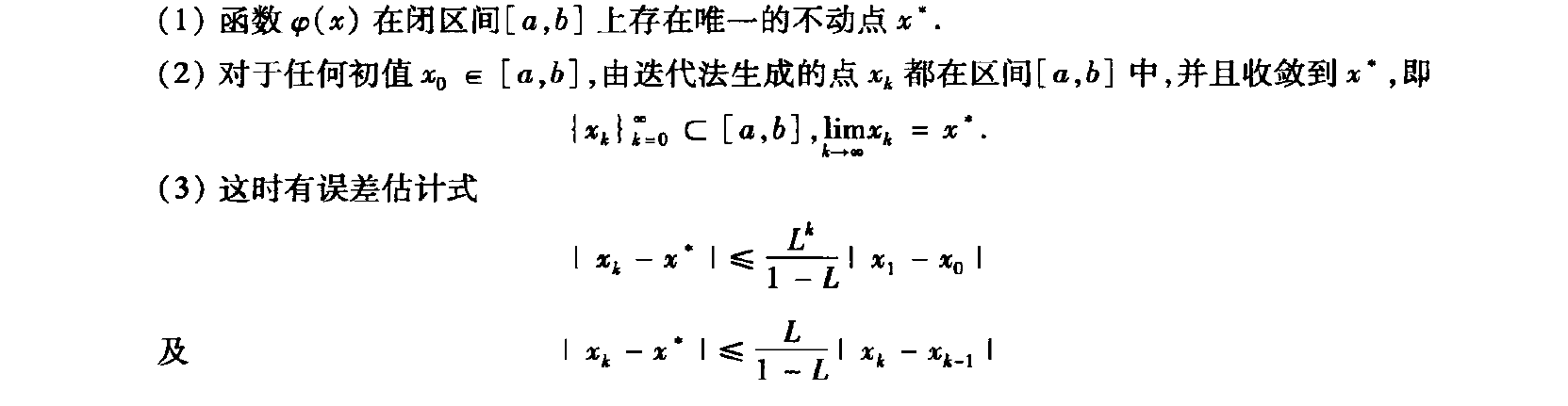三、方程求根的几种常用方法
1、二分法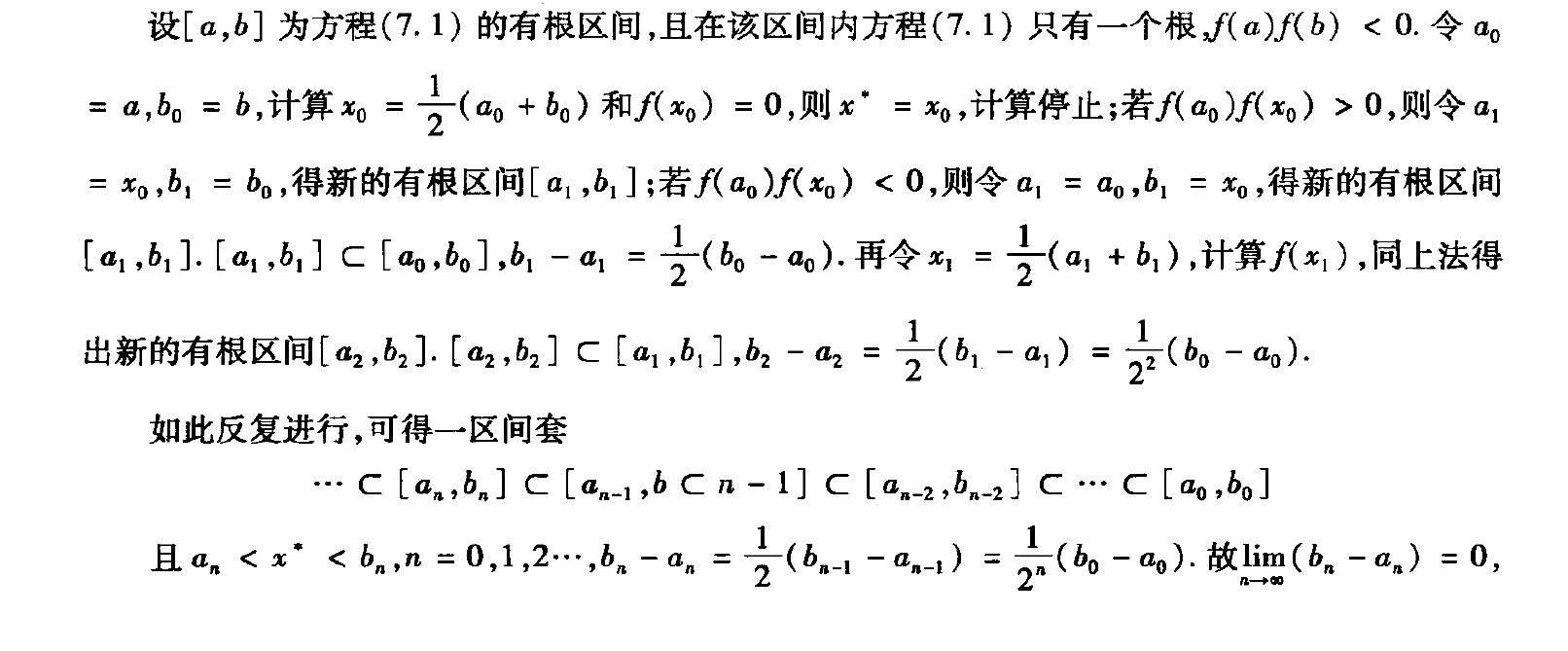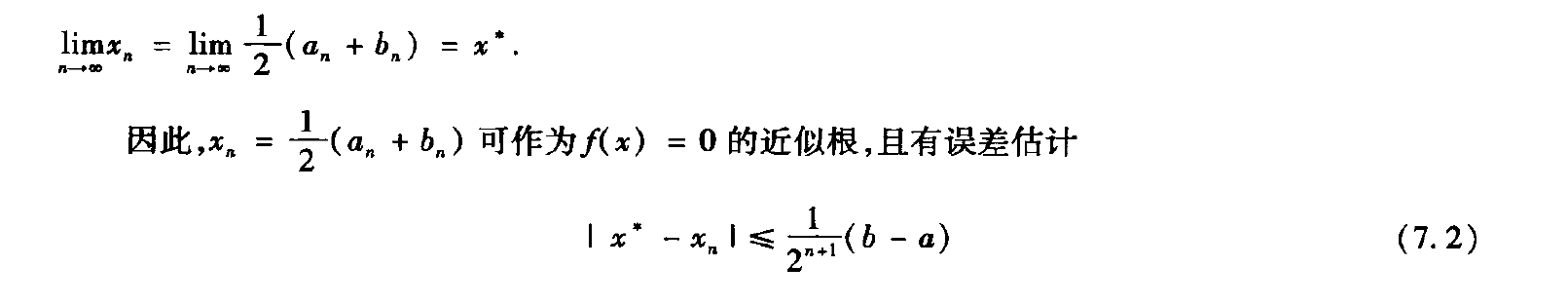2、不动点迭代法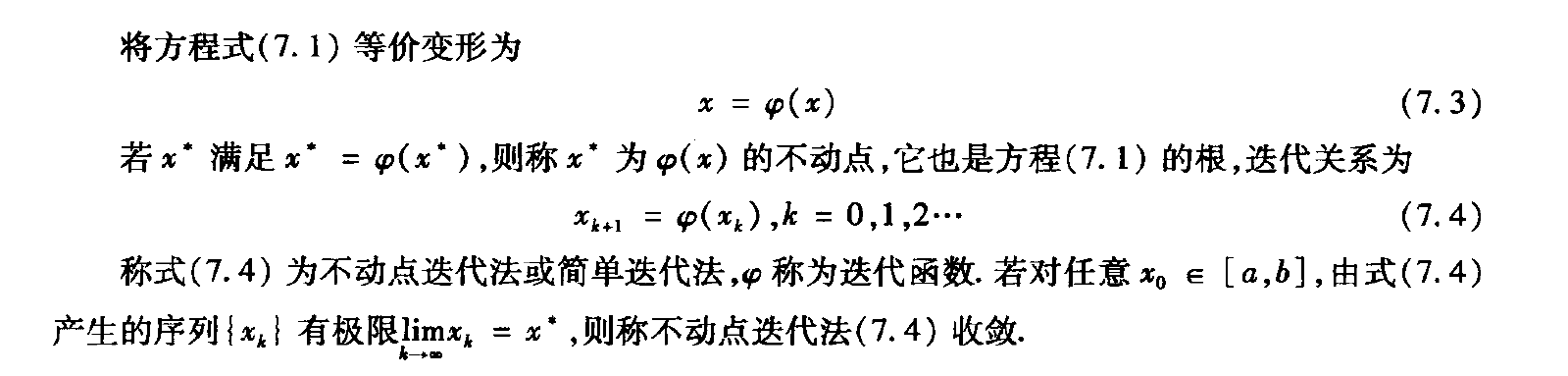3、牛顿法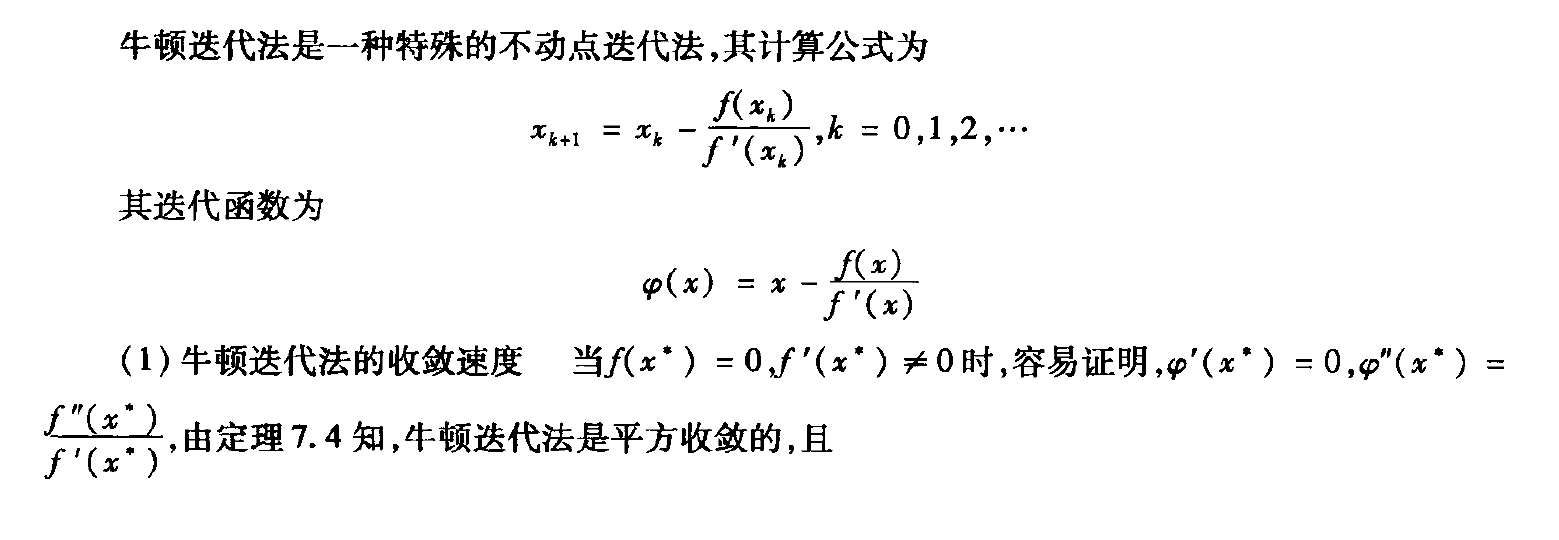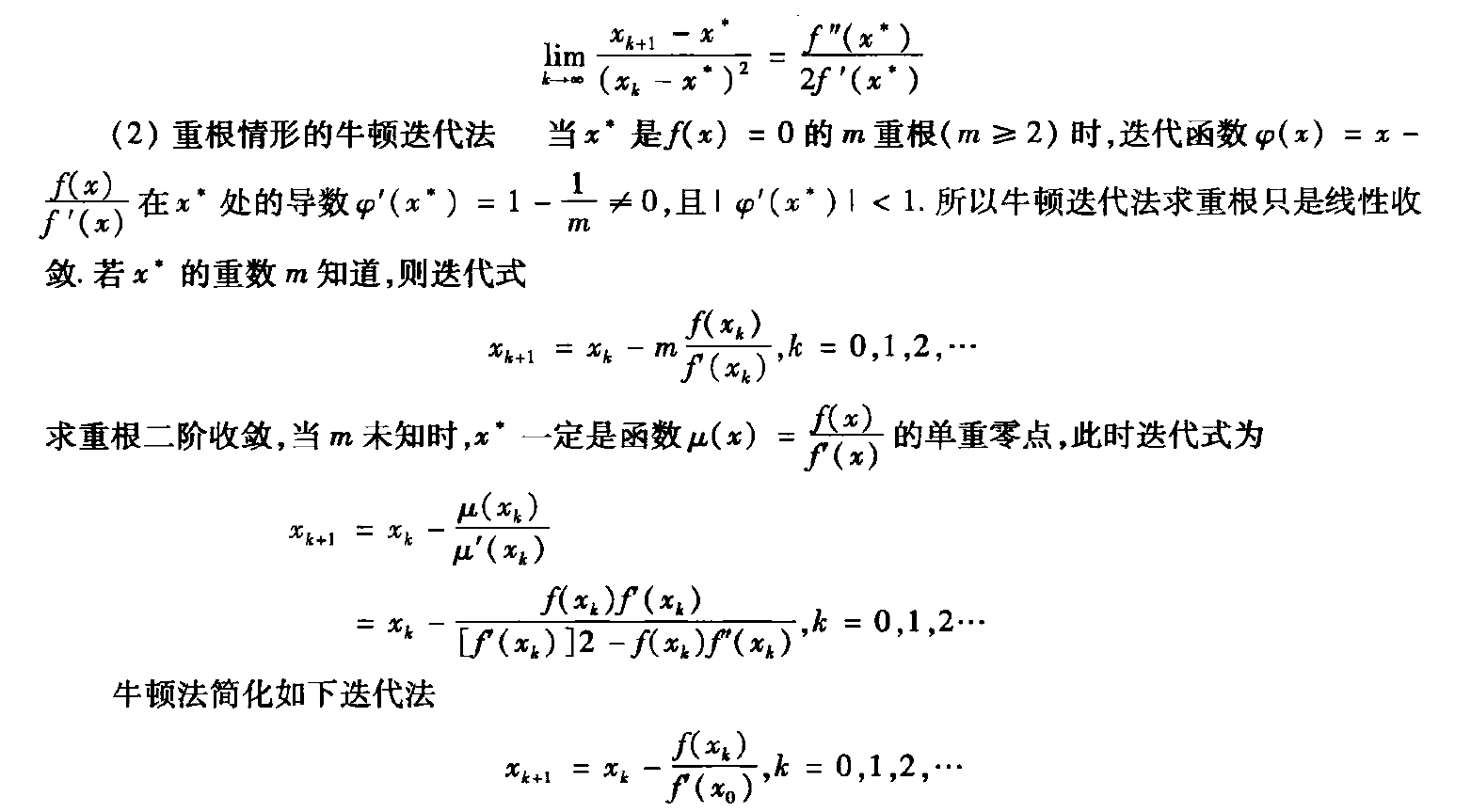题目：编写实现二分法求解方程 x^3-x-1=0与二分法、不动点迭代法、牛顿法求解方程 y=e^x+10x-2=0
代码实现：
import math
import numpy as np
import matplotlib.pyplot as plt
#待求解函数
def f(x):
y=x*x*x-x-1
#y=np.exp(x)+10*x-2
return y
#y=np.exp(x)+10*x-2的一阶导数
def df(x):
y=np.exp(x)+10
return y
#y=np.exp(x)+10*x-2的迭代公式
def phi(x):
y=(2-np.exp(x))/10.0
return y
#二分法
def erfen():
a = input("a = ") #左端点
b = input("b = ") #右端点
c = input("c = ") #预定精度
a = float(a) #强制转换为float类型
b = float(b)
c = float(c)
while(1):
plt.plot([a,b],[f(a),f(b)])
print (a,b)
x=(a+b)/2
if f(x)==0:
break
elif (f(x)*f(a)<0):
b=x
elif (f(x)*f(a)>0):
a=x
elif (a>b):
print ("a,b范围内无解")
if abs(a-b)<c:
break
print ("二分法估计解为：x = ",x)
#不动点迭代法
def FixPoint():
x0 = input("x0 = ")
x0 = float(x0)
espol=0.000001
while(1):
x1 = phi(x0)
print(x1)
if ((np.fabs(x1)<=1) & (float(np.fabs(x1-x0))<espol)):
break
elif ((np.fabs(x1)>=1) & (float(np.fabs(x1-x0)/np.fabs(x1))<espol)):
break
x0=x1
print("不动点迭代法估计解为：x = ", x1)
#牛顿法
def newton():
n=0
x0 = input("x0 = ")
x0 = float(x0)
espol = 0.000001
while (1):
x1 = x0-f(x0)/df(x0)
print(x1)
if ((np.fabs(x1) <= 1) & (float(np.fabs(x1 - x0)) < espol)):
break
elif ((np.fabs(x1) >= 1) & (float(np.fabs(x1 - x0) / np.fabs(x1)) < espol)):
break
x0 = x1
n = n + 1
if (n > 1000):
break

print("牛顿法估计解为：x = ", x1)

#draw
x = np.linspace(0,5,100)  #给定x值的范围
plt.figure(figsize = (7,4)) #设置图的尺寸
plt.plot(x,f(x))

plt.axis([0.5,2,-5,+5])   #设置x，y坐标起始点
# 求解y=x*x*x-x-1时x坐标起始设置为（0.5，2）求解y=np.exp(x)+10*x-2x
坐标起始设置为（0，1）
plt.xlabel("x")
plt.ylabel("y")
plt.title("二分法")
plt.grid(True) #绘制网格
erfen() #调用二分法求解
#FixPoint() #调用不动点迭代法求解
#newton() #调用牛顿法求解
plt.show()




展开全文python 二分法
• 迭代法的作用 许多复杂的求解问题，都可以转换成方程f(x)=0的求解问题。...简单迭代法或基本迭代法又称不动点迭代法 1、不动点（FixedPoint） 首先来看一下什么是不动点： 换句话说，函数φ的不动点是y=φ(x)与y
迭代法的作用
许多复杂的求解问题，都可以转换成方程f(x)=0的求解问题。这一系列的解叫做方程的根。对于非线性方程的求解，在自变量范围内往往有多个解，我们将此变化区域分为多个小的子区间，对每个区间进行分别求解。我们在求解过程中，选取一个近似值或者近似区间，然后运用迭代方法逐步逼近真实解。
方程求根的常用迭代法有：二分法、不动点迭代、牛顿法、弦截法
不动点迭代法
简单迭代法或基本迭代法又称不动点迭代法
1、不动点（FixedPoint）
首先来看一下什么是不动点：换句话说，函数φ的不动点是y=φ(x)与y=x的交点，下图画出了函数y=cos(x)与y=x在区间[0,π/2]的交点，即cos(x)的不动点：2、不动点迭代（Fixed Point Iteration）
不动点迭代的基本思想：也就是说，为了求解方程f(x)=0，首先将方程转换为x=g(x)，然后初始化x0，循环迭代xi+1=g(xi)，直到满足收敛收件。
这里将方程f(x)=0转换为x=g(x)是很容易的，比如对于f(x)=x-cos(x)，求解f(x)=0即为求解x-cos(x)=0，即x=cos(x)，因此g(x)=cos(x)。
再例如对于方程：可以等价为还可以等价为也就是说，将方程f(x)=0转换为x=g(x)有不同的方式，因此对方程f(x)=0来说，g(x)也不是唯一的。
3、不动点迭代的收敛性
这个迭代过程是很简单的，但这里有个关键性的问题：迭代收敛么？即经过N次迭代后是否会收敛于不动点？通俗点讲，若要使不动点迭代收敛，则要求φ(x)在区间[a,b]上的函数值也在此区间内，另外还要求φ(x)的斜率绝对值不大于1。其证明过程比较复杂，有兴趣的可以查阅一些相关文献。
例题
求方程式：x3 - 0.165 × x2 + 3.993 × 10-4 = 0在（0，0.11）上的根
先看看不用迭代法计算的结果
from sympy import *
from sympy.abc import x

def func(x):
return x**3 - 0.165*x**2 + 3.993*10**(-4)
result = solveset(func(x), x, Interval(0, 0.11))
print(result)

结果：
FiniteSet(0.0623775815137495)


约定一个误差，当误差小于某个数值的时候，迭代停止
代码：
xl = 0  #区间下限
xu = 0.11  #区间上限
x = (xl+xu)/2  #迭代初始值
x_list = [x]
i = 0

while True:
x = x ** 3 - 0.165 * x ** 2 + 3.993 * 10 ** (-4) + x
x_list.append(x)
if len(x_list) > 1:
i += 1
error = abs((x_list[-1] - x_list[-2]) / x_list[-1])
if error < 10**(-6):
print(f'迭代第{i}次后，误差小于10^-6')
break
else:
pass

结果：
迭代第777次后，误差小于10^-6
所求方程式的根为0.062370654088088

迭代至电脑默认误差为0
xl = 0  #区间下限
xu = 0.11  #区间上限
x = (xl+xu)/2  #迭代初始值
x_list = [x]
i = 0

while True:
x = x ** 3 - 0.165 * x ** 2 + 3.993 * 10 ** (-4) + x
x_list.append(x)
if len(x_list) > 1:
i += 1
error = abs((x_list[-1] - x_list[-2]) / x_list[-1])
if error == 0:
print(f'迭代第{i}次后，误差为0')
break
else:
pass

print(f'所求方程式的根为{x_list[-1]}')

结果：
迭代第3402次后，误差为0
所求方程式的根为0.062377581513749114

画迭代法
import matplotlib.pyplot as plt
xl = 0  #区间下限
xu = 0.11  #区间上限
x = (xl+xu)/2  #迭代初始值
x_list = [x]
i = 0

x_values = []
y_values = []
while True:
x = x ** 3 - 0.165 * x ** 2 + 3.993 * 10 ** (-4) + x
x_list.append(x)
if len(x_list) > 1:
i += 1
error = abs((x_list[-1] - x_list[-2]) / x_list[-1])
x_values.append(i)
y_values.append(error)
if error == 0:
print(f'迭代第{i}次后，误差为0')
break
else:
pass

print(f'所求方程式的根为{x_list[-1]}')

#设置绘图风格
plt.style.use('ggplot')
#处理中文乱码
plt.rcParams['font.sans-serif'] = ['Microsoft YaHei']
#坐标轴负号的处理
plt.rcParams['axes.unicode_minus']=False
#横坐标是迭代次数
#纵坐标是误差值
plt.plot(x_values,
y_values,
color = 'steelblue', # 折线颜色
marker = 'o', # 折线图中添加圆点
markersize = 1, # 点的大小
)
# 修改x轴和y轴标签
plt.xlabel('迭代次数')
plt.ylabel('误差值')
# 显示图形
plt.show()

结果：
迭代第3402次后，误差为0
所求方程式的根为0.062377581513749114对比牛顿迭代法，牛顿迭代法要快很多，而且准确率也高
牛顿迭代法（Newton’s Method）迭代求根的Python程序


展开全文python
• 非线性方程求解 不动点迭代法 Solution Knowledge 收敛定理一 可以尝试证明一下 1、不动点的存在性 2、不动点的唯一性 3、序列收敛 收敛定理二 这个的条件2更加简洁 可以尝试证明 1、不动点的存在性（其实同定理...
• 1. 不动点和不动点迭代法设 f 是连续函数，为了解方程 把方程变换为等价的方程 其中φ 是连续函数。若x* 是f 的零点，即f(x*)=0 则有 x*称为函数φ 的一个不动点.构造的迭代法 这称为一种...最优化方法
• Matlab 求根代码 包括Newton法、Secant法、Steffenson法、Aitken's法、不动点迭代法，以及包括这些方法求同一个函数时候初始猜测值和迭代次数的比较，并用图像呈现。
• 不动点迭代法的实现 不动点迭代法，是求方程的迭代方法。为什么要迭代的求，直接法不好吗？直接法显然比较好，但是存在弊端，比如函数形式较复杂时，求解器不容易直接求得。利用不动点的性质，可以...
• 1.1.1.不动点与不动点迭代法 对于方程 f(x)=0(1.1)(1.1)f(x)=0f(x) = 0\tag{1.1} 改写成 x=φ(x)(1.2)(1.2)x=φ(x) x = \varphi(x)\tag{1.2} 若要求x∗x∗x^*满足f(x∗)=0f(x∗)=0f(x^*) = 0，则x∗=φ(x∗)x∗...
• 不动点迭代法解非线性方程的newton法matlab实现
• 我的机器学习教程「美团」算法工程师带你入门机器学习 已经开始更新了，欢迎大家订阅~ 任何关于算法、编程、AI行业知识或博客内容的问题，可以随时扫码关注公众号「图灵的猫」，加入”学习小组“，沙雕博主...1、...泛函分析 拓扑 机器学习 统计学习
• 不动点迭代法求方程的根：是迭代法解方程的根当中经典的方法。 将求解f(x) = 0的问题变成x = h(x)这样的问题。 转变的方程很简单，一般是移项，平方，开根号什么的。 难点： 问题难点就得到的对应不动点迭代方程...数值分析 python 数值计算
• 不动点迭代法解非线性方程的aitken加速法matlab实现
• 用MATLAB编写关于如何用史蒂芬不动点迭代法解线性方程组的程序
• 在[a,b]区间内寻找方程x**5-2*x-1=0的根的初始近似值位置，确定不动点迭代的初始点（可能有多个），然后使用不动点迭代法求方程的根（可能有多个根）。前后两次迭代的差的绝对值小于delta后停止迭代。 输入形式 在...python 算法
• 以下介绍方法单独使用时并不实用，只是利用由浅入深的原理形成知识体系。...二、 不动点迭代法 三、牛顿迭代法 牛顿迭代法的实际应用： 四、弦截法 ...二分法
• 对于形如f(x)=0的单变量非线性方程，可以构造不同的迭代函数进行迭代求根，以f(x)=x3-x-1为例，我们可以简单的通过等式变形构造出x...%不动点迭代法1 clc; clear; syms x;%定义变量x f(x)=input('请输入函数表达式,变量matlab
• MATLAB用二分法、不动点迭代法及Newton迭代（切线）法求非线性方程的根 作者：凯鲁嘎吉 - 博客园http://www.cnblogs.com/kailugaji/ 一、实验原理 二、实验步骤 三、实验过程 1.(程序) （1）二分法：求 ...
• 不动点迭代法求方程f(x)=x^3-2*x-1=0的根，按以下两种方案实现，分析迭代函数对收敛性的影响。要求输出每次的迭代结果并统计所用的迭代次数,取精度c=0.5*10^(-5)，x0=2. 方案一：化方程为等价方程x=pow(2*x+1,...c++ 算法
• 分别用二分法、试位不动点迭代、Newton-Raphson和割线求解并比较各方法的收敛速度算法 matlab
• 反之亦然，我们称为函数的一个不动点，求f(x)的零点就等价于求g（x）的不动点，选择一个初始近似值，将其带入右端，即,反复迭代之后得到,g(x)称为迭代函数，而且当k-&gt;∞, 例题： 求方程在附近的根 x=1.5;......